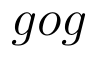Select Page

# 12 Science Maths CBSE Vector Algebra Solutions for MCQ in English

12 Science Maths CBSE Vector Algebra Solutions for MCQ in English to enable students to get Solutions in a narrative video format for the specific question.

Expert Teacher provides 12 Science Maths CBSE Vector Algebra Solutions for MCQ through Video Solutions in English language. This video solution will be useful for students to understand how to write an answer in exam in order to score more marks. This teacher uses a narrative style for a question from Vector Algebra not only to explain the proper method of answering question, but deriving right answer too.

Please find the question below and view the Solution in a narrative video format.

Question:

Solution Video in English:

You can select video Solutions from other languages also. Please check Solutions in ( Hindi )

## Similar Questions from CBSE, 12th Science, Maths, Vector Algebra

Question 1 : Find the unit vector in the direction of the vector(View Answer Video)

Question 2 : Findifand(View Answer Video)

Question 3 : Find the sum of the vectorsand(View Answer Video)

Question 4 : Find the direction cosines of the vector joining the points A(1, 2, -3) and B(-1, -2, 1), directed from A to B. (View Answer Video)

Question 5 : Find a vector in the direction of vectorwhich has magnitude 21 units.  (View Answer Video)

### Differential Equations

Question 1 : Find the particular solution of the differential equation :when x = 1,(View Answer Video)

Question 2 : Solve the differential equation(View Answer Video)

Question 3 : Find the solution of the differential equation(View Answer Video)

Question 4 : Form the differential equation of the family of circles in the second quadrant and touching the co-ordinate axes. (View Answer Video)

Question 5 : Solve the differential equation:(View Answer Video)

### Relations and Functions

Question 1 : Ifis a relation on N, write the range of R. (View Answer Video)

Question 2 : The law a + b = b + a is called ______. (View Answer Video)

Question 3 : Let R be the relation in the set N given by :
R = {(a, b) : a = b - 2, b > 6}.

Question 4 : Letbe defined as. Choose the correct answer. (View Answer Video)

Question 5 : Functionsare defined respectively, by, find(View Answer Video)

### Probability

Question 1 : In a hockey match, both teams A and B scored same number of goals up to the end of the game, so to decide the winner, the referee asked both the captains to throw a die alternately and decided that the team, whose captain gets a six first, will be declared the winner. If the captain of the team A was asked to start, find their respective probabilities of winning the match and state whether the decision of the referee was fair or not.      (View Answer Video)

Question 2 : An experiment succeeds thrice as often as it fails. Find the probability that in the next trials, there will be atleast 3 successes.    (View Answer Video)

Question 3 :  A coin is tossed three times. Find P(F/E), where E : at most two tails and F : atleast one tail.  (View Answer Video)

Question 4 : A pair of dice is thrown 4 times. If getting a doublet is considered a success, find the probability distribution of the number of successes and hence find its mean.  (View Answer Video)

Question 5 : An urn contains 3 white and 6 red balls. Four balls are drawn one by one with replacement from the urn. Find the probability distribution of the number of red balls drawn. Also, find mean and variance of distribution.    (View Answer Video)